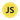# JavaScript Comparison Operators

The basics of JavaScript comparison operators.

In JavaScript, comparison operators are used to checking for equality or differences between values (numbers, strings) and variables. Comparison operators compare operands and returns a boolean value based on whether the value is true or false.

Operands: don’t let this word confuse you. Operands means the values on either side of the comparison operator. Example: `2 < 4`, here `2` and `4` are the operands, and `<` is the less-than operator.

Boolean refers to something that can either be true or false.

## Comparison operator list with description

• `>` greater than
• `<` less than
• `>=` greater than or equal to
• `<=` less than or equal to
• `!=` not equal to
• `!==` not equal value or not equal type
• `==` equal to
• `===` equal value and equal type (strict equality check)

## How to use comparison operators

A simple comparison check using the less than operator:

``````console.log(2 < 4)
// true``````

A strict equality check:

``````console.log(2 === "2")
// false``````

The above returns false because the triple equals `===` operator strictly compares values, including their type. Since we’re comparing a number `2` with a string value type`"2"` it returns false.

Let’s make the same comparison, but this time with equals to (double equals operator) `==`:

``````console.log(2 == "2")
// true``````

This time it returns true because the double equals operator only compares values, not value types.

Comparison operators are also commonly used in conditional statements:

``````const alcoholDrinkingAge = 21
const inputAge = 17

if (inputAge < alcoholDrinkingAge) {
console.log("Sorry you’re too young to drink alcohol")
}``````

Comparison operators are one of the simplest yet most important topics of programming to learn because you’ll use them all the time.

The main thing you need to watch out for in JavaScript is to not confuse the equal to (==) and equal value and equal type (===) operators, because that can get you into some messy situations.

As a rule of thumb, just use the strict triple equals operator `===` and avoid `==`.